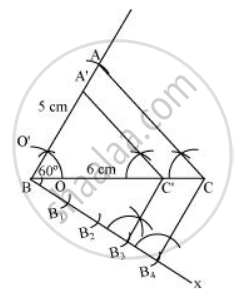Share

# Draw a Triangle Abc with Bc = 6 Cm, Ab = 5 Cm and ∠Abc = 60°. Then Construct a Triangle Whose Sides Are 34 of the Corresponding Sides of the ∆Abc. - CBSE Class 10 - Mathematics

ConceptConstructions Examples and Solutions

#### Question

Draw a triangle ABC with BC = 6 cm, AB = 5 cm and ∠ABC = 60°. Then construct a triangle whose sides are 3/4 of the corresponding sides of the ∆ABC.

#### Solution

A ΔA'BC' whose sides are 3/4of the corresponding sides of ΔABC can be drawn as follows:

Step 1

Draw a ΔABC with side BC = 6 cm, AB = 5 cm and ∠ABC = 60°

Step 2

Draw a ray BX making an acute angle with BC on the opposite side of vertex A.

Step 3

Locate 4 points (as 4 is greater in 3 and 4), B1, B2, B3, B4, on line segment BX.

Step 4

Join B4C and draw a line through B3, parallel to B4C intersecting BC at C'

Step 5

Draw a line through C' parallel to AC intersecting AB at A'. ΔA'BC' is the required triangle.Is there an error in this question or solution?

#### APPEARS IN

Solution Draw a Triangle Abc with Bc = 6 Cm, Ab = 5 Cm and ∠Abc = 60°. Then Construct a Triangle Whose Sides Are 34 of the Corresponding Sides of the ∆Abc. Concept: Constructions Examples and Solutions.
S